# Problem with stylistic set feature

Hi,

I’m trying to setup a stylistic set so that standard numerals will be replaced by special ones:
I setup three lookups:

``````lookup lookup_1 {
sub zero by zero_three.1;
sub one by one_three.1;
sub two by two_three.1;
sub three by three_three.1;
sub four by four_three.1;
sub five by five_three.1;
sub six by six_three.1;
sub seven by seven_three.1;
sub eight by eight_three.1;
sub nine by nine_three.1;
} lookup_1;
lookup lookup_2 {
sub zero by zero_three.2;
sub one by one_three.2;
sub two by two_three.2;
sub three by three_three.2;
sub four by four_three.2;
sub five by five_three.2;
sub six by six_three.2;
sub seven by seven_three.2;
sub eight by eight_three.2;
sub nine by nine_three.2;
} lookup_2;
lookup lookup_3 {
sub zero by zero_three.3;
sub one by one_three.3;
sub two by two_three.3;
sub three by three_three.3;
sub four by four_three.3;
sub five by five_three.3;
sub six by six_three.3;
sub seven by seven_three.3;
sub eight by eight_three.3;
sub nine by nine_three.3;
} lookup_3;

lookup ss030 {
sub Z by y.3;
} ss030;
lookup ss031 {
sub [zero one two three four five six seven eight nine]' lookup lookup_1 ;
sub [zero one two three four five six seven eight nine]' lookup lookup_2 ;
sub [zero one two three four five six seven eight nine]' lookup lookup_3 ;
} ss031;
``````

Then the idea is when inputting e.g. Z123 the output should be /y.3/one_three.1/two_three.2/three_three.1
But when using the feature in Illustrator all three numerals went to the left edge (as if they all got “_three.1”).

See the attached screenshots.

What did I wrong?

Ulrich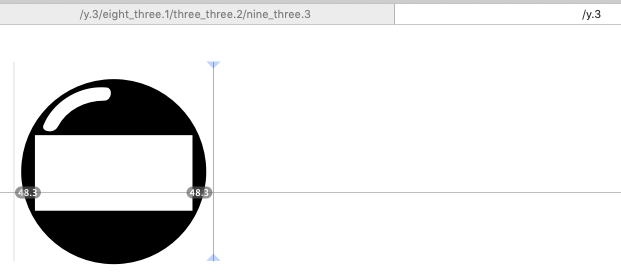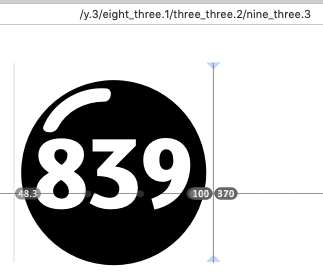But that is what you are doing. The first lookup replaces all figures with `_three.1`. You will need to substitute contextually.

BTW, that is bad glyph naming. The underscore is meant to indicate ligatures. `one_three` is a ligature for 13. Better add all distinguishing features in the dot suffixes. Why not simply call them `one.1`, `one.2` and `one.3`? Or if you need to differentiate between single, double and triple digits, `one.single`, `one.double.1`, `one.triple.1` etc.

O.k., I understand that it was bad glyph naming. Indeed I need what you proposed: one.single, one.double, etc.
What I did not understand is what you mean with “substitute contextually” (I’m a bloody beginner). Can you please give me one example how I can set this up, e.g. for Z123 -> /y.3/one.triple.1/two.triple.2/three.triple.3? That would really help me to understand the mechanism.

Ulrich

I don’t thing you need the .X glyphs. So a one.single, one.double and one.triple might be enough. And you might not even need those, only if they are different in shape or spacing. so maybe you need a .triple because they might need to be narrower.

Everything else can be done be spacing. The glyph that contains the round background has a width that its RSB (right side bearing) is at the left side of the cutout. And the numbers are as wide as their box.

Then you do:

``````sub Z' @figures @figures @figures by Z.triple;
sub Z' @figures @figures by Z.double;
sub Z' @figures by Z.single;
sub Z.triple @figures' by @figuresBlackTriple;
sub @figuresBlackTriple @figures' by @figuresBlackTriple;

sub Z.double @figures' by @figuresBlackDouble;
sub @figuresBlackDouble @figures' by @figuresBlackDouble;
``````

and so one

puuuhh, o.k trying to understand what that meansCan you please comment on mine?!

Do I understand you correctly that the stone (the Z) which in my first attempt had a width of 1000 units should now have a width of RSB? But the cutout of triple, double and single stones are all different.

line 1: If Z is followed by three numerals it is replace by Z.triple
line 2: If Z is followed by two numerals it is replace by Z.double
line 3: If Z is followed by one numeral it is replace by Z.single
line 4: If Z.triple is followed by a numeral that one is replaced by figuresBlackTriple
line 5: If figuresBlackTriple is followed by a numeral that one is replaced by figuresBlackTriple
line 6: If Z.double is followed by a numeral that one is replaced by figuresBlackDouble
line 7: If figuresBlackDouble is followed by a numeral that one is replaced by figuresBlackDouble

Afer line 5: what about the third numeral?
What are in fact Z.triple, Z.double, Z.single, figuresBlackTriple, figuresBlackDouble, figuresBlackSingle?
Is Z.triple the round stone with a cutout for three numerals, etc.?
But what are figuresBlackTriple etc.?

Sorry for being so slow-witted.

Is there a fundamental design difference between single, double or triple figures? Like, are the singles and doubles wider than the triples? If not, then you do not need to differentiate between them, and just take care of their positioning.

``````lookup replaceZ {
sub Z' @figures @figures @figures by Z.triple;
sub Z' @figures @figures by Z.double;
sub Z' @figures by Z.single;
} replaceZ;
``````

And then continue with the figures thereafter:

``````lookup replaceFiguresAfterZ {
sub Z.triple @figures @figures @figures' by @figuresMini;
sub [Z.double Z.triple] @figures @figures' by @figuresMini;
sub [Z.single Z.double Z.triple] @figures' by @figuresMini;
} replaceFiguresAfterZ;
``````

Then all you need to do is add kerning to Z.triple with the mini figures. Make sure they all have the same kerning groups. Or you could add that as an additional, positional lookup:

``````lookup moveMiniFigures {
pos Z.triple @figuresMini -500;
pos Z.double @figuresMini -450;
pos Z.singl @figuresMini -400;
} moveMiniFigures;
``````

But you cannot preview positional lookups inside Glyphs, so simple kerning may be the more workable choice.

No the figures are the same, only the position varies.
I’ve tried your solution but something still went wrong.
figures: one two three four five six seven eight nine zero
figuresMini: one two three four five six seven eight nine zero
(I don’t understand what the purpose of figuresMini are)

In Illustrator when I use ss03 and type Z222 the placement was wrong (first screenshot), when only two or one numerals are typed the went off the circle (screenshot 2 & 3).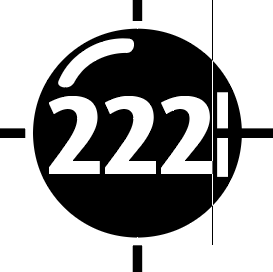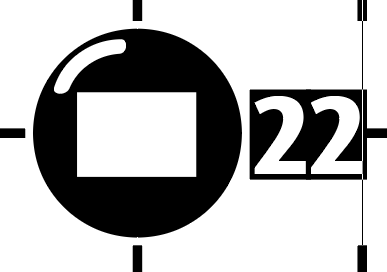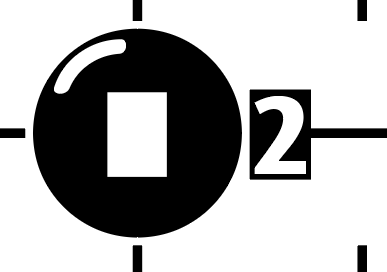Of course you need to adjust the kern values, `-500`, `-450` and `-400` were just examples.

The figures small enough for fitting into the circle, the ones you show in the samples. I do not know what your regular ones look like. So now I am not sure what exactly the screenshots are showing. Can you send me the .glyphs file?

I mean set the width of the Z glyphs so that the RSB is at the left edge of the cut out. So that the next glyph will be inside it. The RSB will be quite a bit negative then.

OK I learned that AI ignores GPOS features if they are in a stylistic set or in stylistic alternates.

And now I understand, your default figures are already the small figures. I didn’t figure that one out. Go figure.

So, here is a setup that works:

``````lookup replaceZ {
sub Z' @figures @figures @figures by Z.triple;
sub Z' @figures @figures by Z.double;
sub Z' @figures by Z.single;
} replaceZ;

lookup moveFigures {
pos Z.triple @figures' <-900 0 -900 0> @figures' <0 0 0 0> @figures' <0 0 90 0>;
pos Z.double @figures' <-770 0 -770 0> @figures' <0 0 230 0>;
pos Z.single @figures' <-635 0 -270 0>;
} moveFigures;
``````

For this to work, I gave the Z circles normal (positive) sidebearings again. The quadruple figures mean: . Basically, move is the movement of the shape, and advance is the following cursor position. The way I arranged them is so that the figures are moved into the circle and the following cursor will always be after the circle.

Wherever you put this (mind you, stylistic sets have only been available in AI since version 2019, so you may want to do that in a different feature, e.g. contextual or stylistic alternates), repeat the GPOS lookup `moveFigures` in `kern`, like this:

You can repeat lookups more often, they will always be effective only once. You do not need to worry that the figures will be moved twice.

I will send you the file back in DM.

Thank you very very much!
I’m still irritated. In Illustrator this does not work. I do not get the white stone variants and also the black stones with figures are not placed correctly. How did you type them?
I then made a test in InDesign and there everything seems to work! For my book project it doesn’t bother me because it is typeset in InDesign. But in general it should work also in Illustrator. Mmmh black magic.

That is not black magic but the shitty Opentype support in Adobes apps.

Which version of AI are you using? Only the latest version supports ssXX. And you do need to activate Contextual Alternates in AI’s OpenType palette. But you can try moving the code in other features that may be always on in AI (rlig? ccmp? locl?). You can move the lookup definitions into the prefix, and repeat the lookup calls many times in different features.

But I am not sure about AI’s OT support. It is generally not so good, as Georg wrote. Contextual Alternates, for example, should be on by default.

I’m using v2019.
The wrong placement was my fault because in the version I sent you the “normal” figures had a width of 250 instead of 270 (like the whit on black ones).
But I cannot get a white stone with figures in Illustrator. In InDesign it works.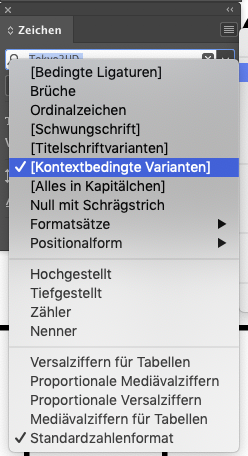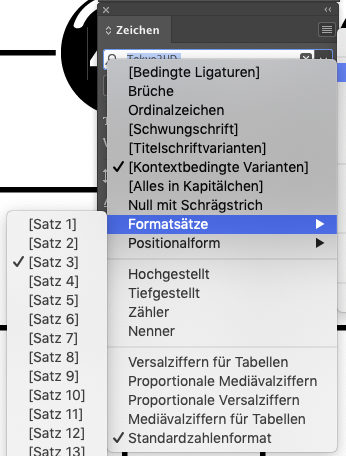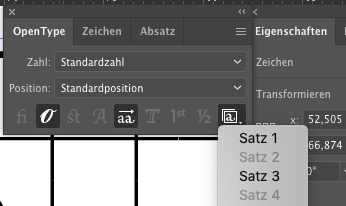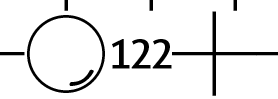The last two screenshots are from Illustrator the others from InDesign.

I got another question.

I have variants of the same stone: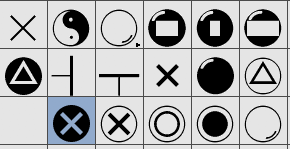I would like to have the possibility to select the stone in InDesign via the little triangle (all the stones with triangles, crosses and circles inside). I thought I have to define the feature “calt” for this purpose. But I failed:
`sub W from [W.1 W.2 W.3 W.4];`
The “W” did not get this triangle for selecting alternate glyphs.

Ah, I got it – missed to refresh the “aalt”.

1 Like

I had to rename some glyphs and add another OT feature, take a close look at the file I sent you.